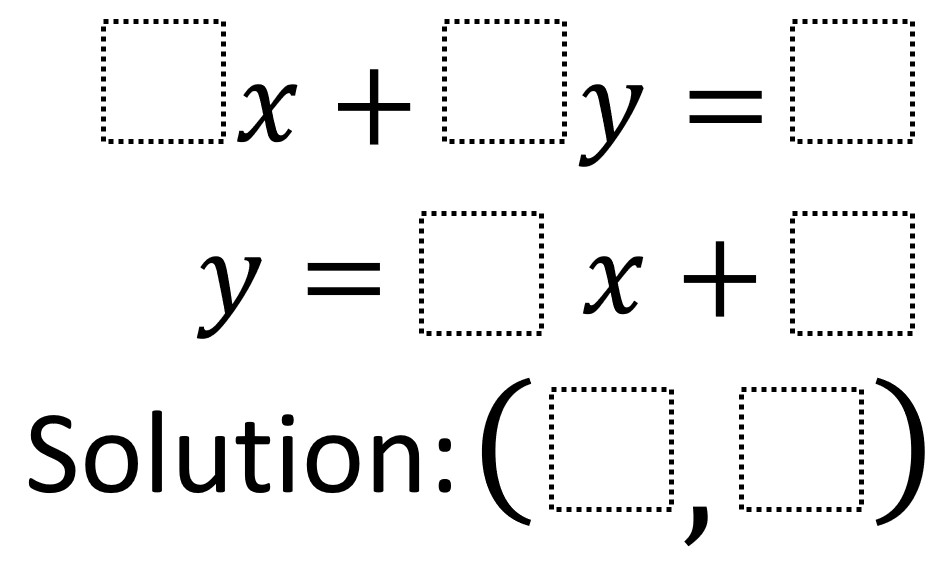# Systems of Equations 4

Directions: Using the integers −9 to 9 at most one time each, place an integer in each box to create a system of equations whose solution is as close to the origin as possible.### Hint

How can you check whether your lines intersect at your solution algebraically? How can you check whether your lines intersect at your solution graphically?

-1x + 3y = 5
y = 4x + -2
Solution: (1, 2)

Source: Robert Kaplinsky

## Difference of Squares and Sum of Cubes

Directions: Using the digits 0 to 9 at most one time each, place a digit …

1.2.